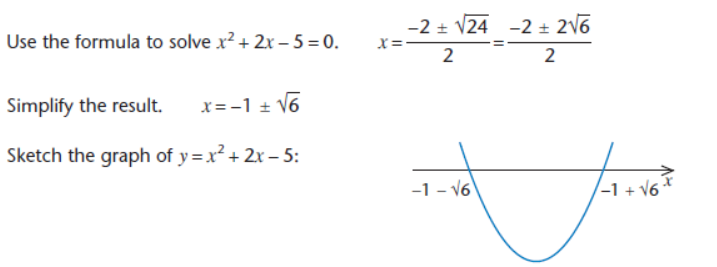## Inequalities

When dealing with inequalities, it is important to remember that if you divide or multiply by a negative number, the direction of the inequality is changed.

When solving quadratic inequalities (inequalities with x2 in them), it is necessary to analyse the various cases to solve the inequality.

Example

Solve x2 + 3x + 2 > 0

Now, we can factorise the left hand side to get:

(x + 2)(x + 1) > 0

To solve this, we need to divide it up into a number of cases, based upon when the sign of one of the factors changes:

 x < -2 -2 < x < -1 x > -1 (x + 2) negative positive positive (x + 1) negative negative positive (x + 2)(x + 1) positive negative positive

So when x < -2, for example, we know that (x + 2) is less than 0 and (x + 1) is less than zero. Therefore (x + 2)(x + 1) > 0 (since negative times negative = positive).

From the table, therefore, (x + 2)(x + 1) > 0 when x < -2 or x > -1 .

Drawing a table like this makes things easier.

Alternatively, you can draw the graph of x2 + 3x + 2 > 0 and look at where the graph lies above the x-axis. You should get the same answer.

Linear Inequalities

Linear Inequalities can be solved by rearrangement in much the same way as linear equations. You must make sure however that you reverse the direction of the inequality when dividing or multiplying by a negative.

Example

Solve the inequality                                                             8 – 3x > 23.

Subtract 8 from both sides of the equation:                      -3x > 15.

Divide both sides of the equation by -3:                                x < -5.

An Inequality which has x on both sides is treated like a corresponding equation.

Example

Solve the inequality                         5x – 3 > 3x – 10.

Subtract 3x from both sides:           2x – 3 > - 10.

Add 3 to both sides:                               2x > -7.

Divide both sides by 2:                             x > - 3.5